# Introduction to the Financial Time Series Analysis using Pandas - Part 1¶

As defined in Investopedia A time series is a sequence of numerical data points in successive order. Generally, observation points are successively equally spaced in time. Most of the financial data is in the time series format and hence "Financial Time Series Analysis" is an important tool for anyone trying to understand the historical movements, predict the future movements or manage the risk associated with the future movements.

Through this tutorial, we are providing tools that are neccessary to get access to the financial data and perform a preliminary analysis and visualization to understand the data better.

By the end of this tutorial you will be capable of doing the following tasks:

• Load data of any financial instrument using Quandl's Python package

2. Plot Time Series data

• Plot the stock price data
• Plot subplot for price and volume traded
• Normalize the time series to see how your investment grew

3. Returns Calculation

• Calculate simple return
• Calculate and plot the daily returns
• Calculate the Compound Annual Growth Rate(CAGR)

4. Volatility Calculation

• Calculate the annualized volatility
• Calculate and plot the rolling volatilty
• Relationship between Vol and returns

5. Correlation Calculation

• Generate pair-wise plot and analyse
• Generate correlation metrics

6. Returns Distribution and Analysis

• Plot the return distribution
• Normality test

7. Monthly plot

• Comment on seasonality

8. Digging deep

• Calculate and plot the moving average
• Perform the drawdown analysis

In this tutorial we are using Quandl's free API for sourcing financial data. The pre-requisites for using Quandl API are:

1. Register on Quandl and generate API key.
2. Install Quandl package in python and import the same.

### Importing Packages¶

Before jumping into the actual code, the first step is to import all the packages that will be used in this tutorial.

In :
import quandl as qd #Quandl for downloading financial data
import numpy as np #Numpy for access to arrays and vectorization of calculaitons
import matplotlib.pyplot as plt #Matplotlib for pythons basic plotting
import seaborn as sns #Seaborn for heatmap plotting
import scipy.stats as stats #Scipy Stats module for access to statistical formula.
import plotly
import plotly.plotly as py
import plotly.graph_objs as go
import seaborn as sns
import pylab
import scipy.stats as stats
import warnings
from datetime import date
warnings.filterwarnings('ignore')
plt.style.use('ggplot')#For chart formating we have set in-build formating convention


### Load live data of any financial instrument using Quandl's Python package.¶

The data can be downloaded by specifying following parameters to the get function of Quandl

• Ticker for which the data is required
• Start date for the time series
• End date for the time series
• Quandl API key for aunthentication

The data will be downloaded as a pandas DataFrame, which will have the dates as index and market data such as Open, High, Low, Close prices, Shares Traded and Turnover (Rs. Cr) as columns.

We can print first 5 rows of our data by using "head" function. This helps us to have a quick look at the structure of the data downloaded.

In :
authtoken = "dhpDwSsxjAu6XDfnVTjd"
ticker = "NSE/NIFTY_50"
start_date = "2010-01-01"
end_date = "2018-06-30"
nifty_levels_test = qd.get(ticker, start_date=start_date, end_date=end_date, authtoken=authtoken)

               Open     High      Low    Close  Shares Traded  \
Date
2010-01-04  5200.90  5238.45  5167.10  5232.20    148652424.0
2010-01-05  5277.15  5288.35  5242.40  5277.90    240844424.0
2010-01-06  5278.15  5310.85  5260.05  5281.80    216147837.0
2010-01-07  5281.80  5302.55  5244.75  5263.10    181246734.0
2010-01-08  5264.25  5276.75  5234.70  5244.75    201910800.0

Turnover (Rs. Cr)
Date
2010-01-04            6531.61
2010-01-05            7969.62
2010-01-06            7892.60
2010-01-07            6890.99
2010-01-08            7777.04


info function gives list of column names in pandas dataframe, their data type and number of non 'NaN' values.
describe function provides basic statistics such as count, mean, std, min/max etc. for each column

In :
print("***************Basic information about data downloaded***************")
print(nifty_levels_test.info())
print("***************Data description***************")
print(nifty_levels_test.describe())

***************Basic information about data downloaded***************
<class 'pandas.core.frame.DataFrame'>
DatetimeIndex: 2111 entries, 2010-01-04 to 2018-06-29
Data columns (total 6 columns):
Open                 2111 non-null float64
High                 2111 non-null float64
Low                  2111 non-null float64
Close                2111 non-null float64
Turnover (Rs. Cr)    2093 non-null float64
dtypes: float64(6)
memory usage: 115.4 KB
None
***************Data description***************
Open          High           Low         Close  Shares Traded  \
count   2111.000000   2111.000000   2111.000000   2111.000000   2.093000e+03
mean    7135.990881   7172.192492   7090.158361   7131.309332   1.727730e+08
std     1779.522933   1779.082316   1775.820220   1777.239695   5.805946e+07
min     4623.150000   4623.150000   4531.150000   4544.200000   6.555703e+06
25%     5530.525000   5564.650000   5486.650000   5530.825000   1.353542e+08
50%     6530.000000   6562.850000   6497.650000   6526.650000   1.628396e+08
75%     8470.825000   8505.850000   8423.425000   8471.825000   1.989305e+08
max    11120.850000  11171.550000  11075.950000  11130.400000   6.291986e+08

Turnover (Rs. Cr)
count        2093.000000
mean         7656.236885
std          2854.432124
min           297.890000
25%          5782.230000
50%          7105.680000
75%          8920.290000
max         29479.770000


## 2. Ploting Time Series data¶

The first step to understand and analyse financial data is through visualization. By simply ploting the financial timeseries we can get the understanding of how an investment has performed over the time period.

### Plot the Stock Price data¶

The code given below uses the package matplotlib.pyplot to plot the 'Close', 'High' and 'Low' columns of the time series downloaded for NIFTY50 as a line graph. We can customize the chart to show its title, the label for the y axis and its legend.

In :
plt.rcParams['figure.figsize'] = [12, 8]
_ = nifty_levels_test[['Close','High','Low']].plot()
plt.ylabel('Index Level')
plt.title (ticker +' LEVEL TIME SERIES')
plt.legend()
plt.show()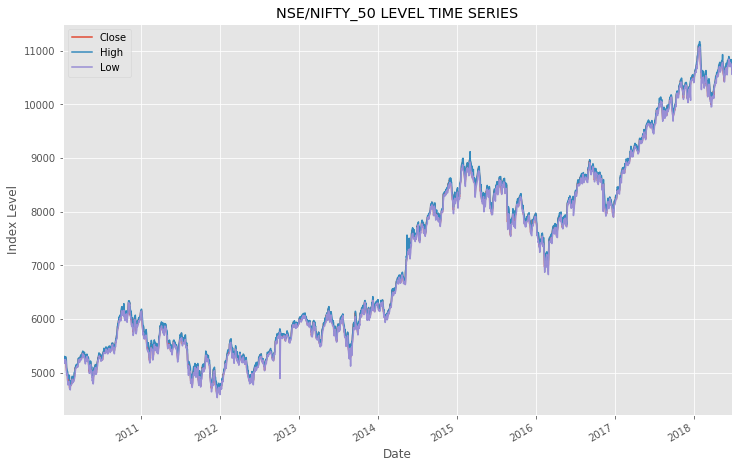### Plot Subplot for Price and Volume traded¶

The code given below subsets the timeseries downloaded for NIFTY50 for dates greater than 2017-12-31 and handles the missing values by using the forward fill method. Then it creates 2 subplots, one of which is the line chart for 'Close', 'High' and 'Low', while the second is the line chart for the volume of 'Shares Traded'. Looking at these graphs adjacent to each other helps us in observing the relationship between price movement and volumen traded.

In :
slice_start_date = pd.datetime(2017,12,31)
nifty_levels_test_sliced = nifty_levels_test[nifty_levels_test.index > slice_start_date].fillna(method='ffill')
f, (a0, a1) = plt.subplots(2,1, gridspec_kw = {'height_ratios':[3, 1]})
_ = nifty_levels_test_sliced[['Close','High','Low']].plot(ax=a0)
a0.set_ylabel('Index Level')
a0.grid(True)
a0.set_xticklabels([])
x_label = a0.set_xlabel('')
x_label.set_visible(False)
a0.set_title (ticker +' LEVEL TIME SERIES (Sliced)')
a0.legend()
a1.set_ylabel('Volume')
f.tight_layout()
plt.show()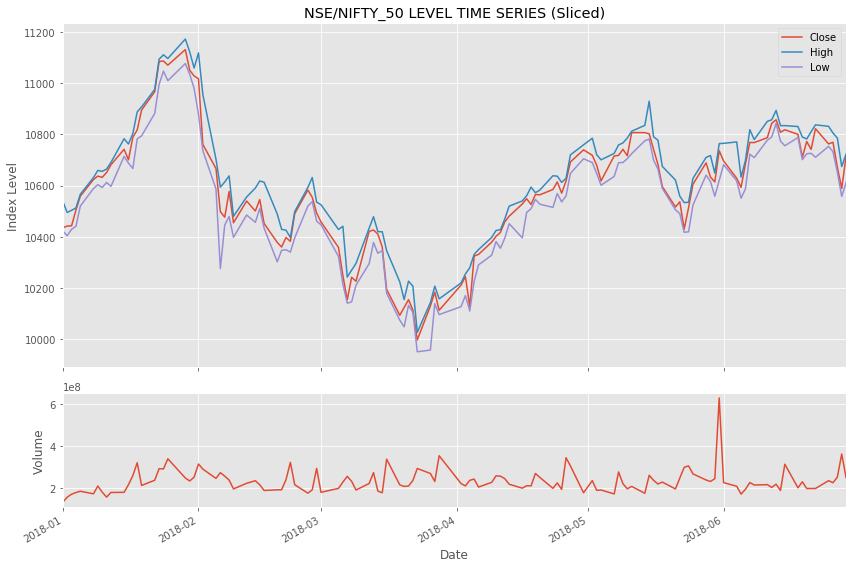### Normalize the Time Series¶

A financial time series is normalized to observe how an investment of x amount changes with time. It is useful for comparing the performance of multiple time series.

Normalization is done by using the formula given below:

\begin{equation*} \frac{p(t)}{p(0)} * x \quad\quad \text{for $0<=t<=T$ where T is period end date} \end{equation*}

The code given below normalizes and plots the sliced time series to see how an investment of 1000 made in NIFTY50 on 2017-12-31 changes over time.

In :
nifty_close_levels = nifty_levels_test_sliced['Close']
normalised_start_level = 1000
nifty_close_normalized = nifty_close_levels/nifty_close_levels*normalised_start_level
_ = nifty_close_normalized.plot()
plt.ylabel('Index Level')
plt.title (ticker +' LEVEL TIME SERIES (Normalized)')
plt.legend()
plt.show()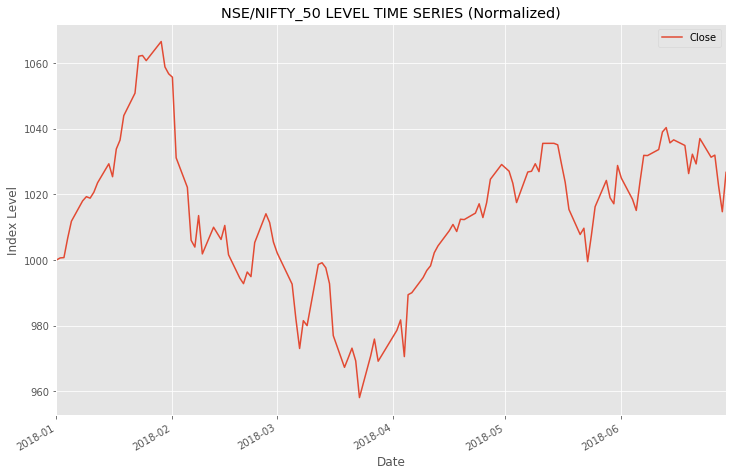We will try to repeat the above process but this time with multiple stocks

In :
stock_codes = stock_codes =["GM", "F", "AAPL", "MSFT"]
start_date_multi = '2011-12-31'
source = 'WIKI/PRICES'
stock_prices = qd.get_table(source, qopts = { 'columns': ['ticker', 'date', 'adj_close'] }, ticker = stock_codes, date = { 'gte': start_date_multi, 'lte': end_date })

     ticker       date  adj_close
None
0      MSFT 2018-03-27      89.47
1      MSFT 2018-03-26      93.78
2      MSFT 2018-03-23      87.18
3      MSFT 2018-03-22      89.79
4      MSFT 2018-03-21      92.48


We need to process above dataframe further to get desired format

In :
stock_prices_formatted = stock_prices.pivot(columns = 'ticker', index='date', values='adj_close')

Out:
ticker AAPL F GM MSFT
date
2012-01-03 52.848787 8.772734 18.035831 22.792249
2012-01-04 53.132802 8.906729 18.121512 23.332995
2012-01-05 53.722681 9.135309 18.995457 23.571435
2012-01-06 54.284287 9.229893 19.638064 23.933352
2012-01-09 54.198183 9.300832 19.569519 23.622529

This time we will try Plotly to plot interactive plots.

In :
data = [go.Scatter(x=stock_prices_formatted.index, y=stock_prices_formatted[udl_code], name=udl_code)
for udl_code in stock_prices_formatted.columns]
layout = dict(
title = "Price Time Series")

fig = dict(data=data, layout=layout)
py.iplot(fig, filename = 'Price Time Series')

Out:

Its difficult to compare the performane of different underlying give they all are trading at different levels. Normalisation as discussed above will help to compare performance of these 4 stocks.

In :
norm_stock_prices_formatted = stock_prices_formatted/stock_prices_formatted.iloc[0,:]*100
data = [go.Scatter(x=norm_stock_prices_formatted.index, y=norm_stock_prices_formatted[udl_code], name=udl_code)
for udl_code in norm_stock_prices_formatted.columns]
layout = dict(
title = "Normalized Price Time Series")

fig = dict(data=data, layout=layout)
py.iplot(fig, filename = 'Normalized Price Time Series')

Out:

## 3. Returns Calculation¶

The return of a financial instrument is the profit/loss incurred on an investment over a period of time, expressed as a proportion of the original investment. It gives a complete, scale-free summary of the investment opportunity.

The simple single period return of financial instrument is calculated using the formula given below

\begin{equation*} R(t) = \frac{P(f)}{P(i)} -1 \quad\text{where P(i) and P(f) are the prices at the starting time point and ending time point of the period} \end{equation*}

The code given below calculates the simple return for the period between 2017-12-31 and 2018-06-30

In :
nifty_simple_return = (nifty_close_levels[-1]/nifty_close_levels -1)
print("Nifty returns for period starting from {} till {} is {:.2f}%".format\
(slice_start_date.date(),end_date, nifty_simple_return*100))

Nifty returns for period starting from 2017-12-31 till 2018-06-30 is 2.67%


### Simple Daily Return¶

The daily return is the day over day return.

The underlying formula for calculating simple daily return is

\begin{equation*} R(t) = \left( \frac{P(t)}{P(t-1)} -1 \right) \quad\text{for $0<=t<=T$ } \end{equation*}

The code given below calculates the daily returns using the pandas dataframe function pct_change. We also calculated the mean daily simple return, which comes out to be close to zero. This tells the importance of compounding effect.

The return series hence obtained is first plotted as a line chart and then as a histogram to observe its underlying distribution. From histogram we can observe that return distribution is bell shaped and similar to famous normal distribution.

In :
daily_returns = nifty_levels_test['Close'].pct_change()
print("Avg daily return is {:.6f}%".format(daily_returns.mean()*100))
_ = daily_returns.plot()
plt.ylabel('Index Daily Returns')
plt.title (ticker +' RETURN TIME SERIES')
plt.legend()
plt.rcParams['figure.figsize'] = [12, 8]
plt.show()
_1 = daily_returns.hist(bins=30)
plt.title('Daily return distribution')
plt.show()

Avg daily return is 0.038790%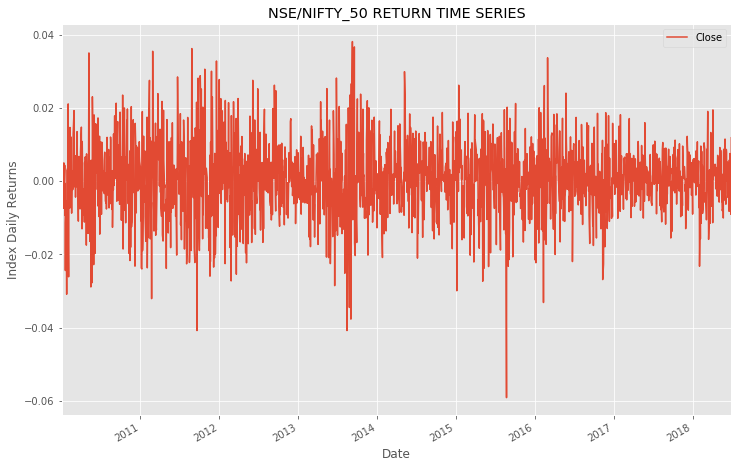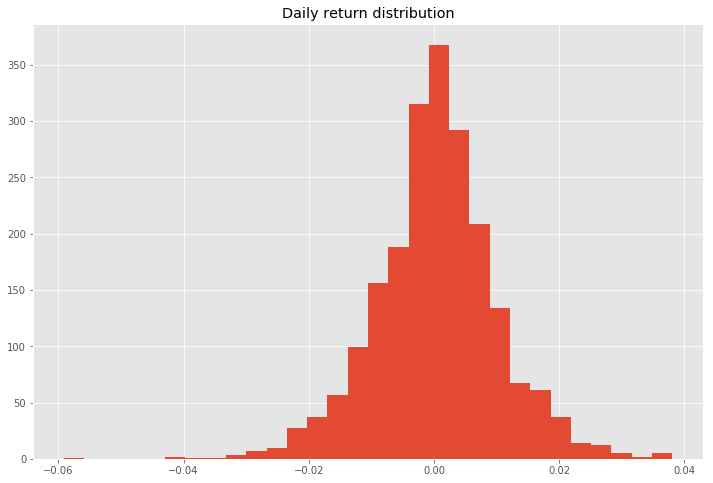### Logarithmic Return / Continuously Compounded Return¶

The logarithmic return or continuously compounded return, D(t), is calculated using the formula

The advantages of using log returns over simple returns are as follows:

1. The logarithmic returns are symmetric in nature, as opposed to simple returns.
2. In case the prices are distributed log-normally, log(1+R(t)) is distributed normally.
3. The log returns are additive in nature.

The code given below calculates the log returns using the log function from the numpy library. Instead of calculating log returns from scratch, we have used simple returns timeseries to calculate log returns.

The returns hence obtained are plotted as a histogram to observe the distribution of these returns. As we can observe the log returns distribution is also bell shaped and similar to normal distibution.

In :
daily_log_returns = np.log(daily_returns+1)
_1 = daily_log_returns.hist(bins=30)
plt.title('Daily log return distribution')
plt.show()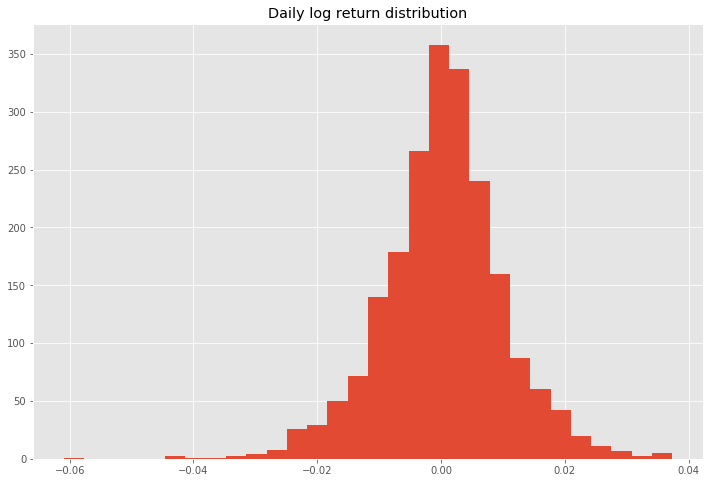### Compound Annual Growth Rate (CAGR)¶

The Compound Annual Growth Rate (CAGR) is the mean annual growth rate of an investment over a specified period of time longer than one year. It describes the rate at which an investment would have grown, if it had grown at a steady rate, which doesn't ususally happen in reality. CAGR can be essentially viewed as a way to smooth out an investment’s returns so that they may be more easily understood.

The formula for calculating CAGR is

\begin{equation*} R(t) = \left( \frac{P(f)}{P(i)}\right)^\left( \frac{1}{n}\right) -1 \quad\quad\text{where P(f) and P(i) are the final and the initial values for a period and n is the time in years } \end{equation*}

CAGR is a relatively simple metric and hence has a variety of uses.

1. CAGR can be used to calculate the average growth of a single investment
2. It can be used to compare investments of different types with one another. For example it can be used to compare an investment in risk free instrument with an investment in a portfolio with varying growth rate
3. It can also be used to track the performance of various business measures of one or multiple companies alongside one another

The code given below illustrates how CAGR can be calculated.

In :
time_in_years = (nifty_levels_test.index[-1] - nifty_levels_test.index).days/365.0
cagr = (nifty_levels_test['Close'][-1]/nifty_levels_test['Close'])**(1/time_in_years) - 1
print("Compound annual growth rate from {} to {} for {} is {:.2f}%".format\
(nifty_levels_test.index.date(), nifty_levels_test.index[-1].date(), ticker, cagr*100))

Compound annual growth rate from 2010-01-04 to 2018-06-29 for NSE/NIFTY_50 is 8.81%


## 4. Volatility Calculation¶

### Annualized Volatility¶

Annualised volatility is the degree of variation observed in the prices of a financial instrument over a period of one year. It can be calculated by scaling the daily volatility, which is measured as the standard deviation of the returns of the stock prices, to 1 year. The daily volatility can be scaled to annual by simply multiplying with $\sqrt{252}$ (considering that there are 252 business days in a year) in case it is calculated as the standard deviation of the log returns.

The code given below calcluates annualised volatility by calculating the standard deviation of log returns of NIFTY50 using pandas dataframe function std and then multiplying it by $\sqrt{252}$.

In :
volatility = daily_log_returns.std() * (252**0.5)
print("Annualized daily volatility from {} to {} for {} is {:.2f}%".format\
(nifty_levels_test.index.date(), nifty_levels_test.index[-1].date(), ticker, volatility*100))

Annualized daily volatility from 2010-01-04 to 2018-06-29 for NSE/NIFTY_50 is 15.58%


### N-days Rolling Volatility¶

N-days rolling volatility is calculated by rolling the dates for a window of N days and using the corresponding data for calculating the volatility.

The code given below calculates the rolling annualized volatility for NIFTY50 for a window of 252 days using pandas function rolling. The series of rolling volatility hence obtained is plotted on the secondary y-axis, along with index levels plotted on the primary y-axis.

In :
rolling_volatility = daily_log_returns.rolling(window = 252).std()*(252**0.5)*100
nifty_levels_test['Close'].plot(label='Index Levels', color='Blue')
plt.ylabel(ticker + ' levels')
plt.legend()
ax = plt.gca()
ax2 = ax.twinx()
ax2.set_ylim(ymax=50)
rolling_volatility.plot(label='Volatility')
plt.title(ticker +" levels and 252 days Rolling Volatility")
plt.ylabel("Volatility in %")
plt.legend()
plt.show()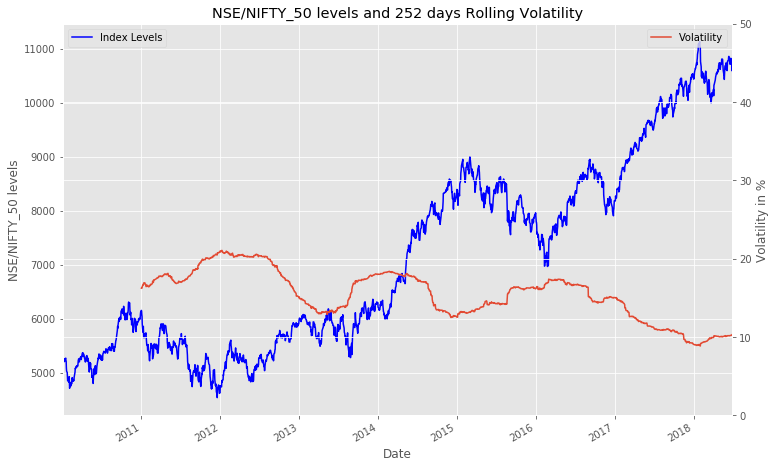Studying correlation between two stocks

## 5. Correlation Calculation¶

In :
log_returns = np.log(norm_stock_prices_formatted.pct_change().dropna()+1)

Out:
ticker AAPL F GM MSFT
date
2012-01-04 0.005360 0.015159 0.004739 0.023448
2012-01-05 0.011041 0.025340 0.047100 0.010167
2012-01-06 0.010400 0.010301 0.033270 0.015237
2012-01-09 -0.001587 0.007656 -0.003497 -0.013072
2012-01-10 0.003574 0.000000 0.017362 0.003598
In :
g = sns.pairplot(log_returns, diag_kind="kde")
plt.show()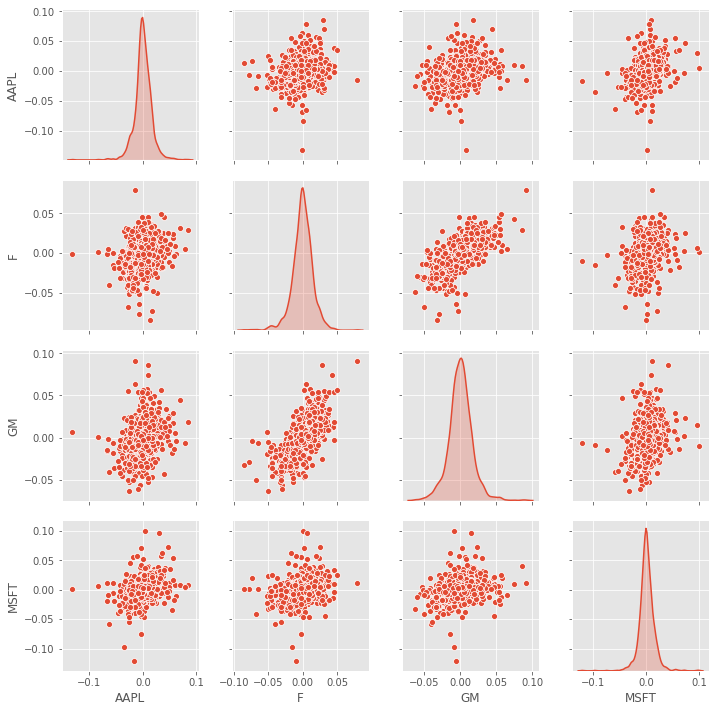In :
log_returns.corr()

Out:
ticker AAPL F GM MSFT
ticker
AAPL 1.000000 0.295965 0.282291 0.356120
F 0.295965 1.000000 0.692737 0.329482
GM 0.282291 0.692737 1.000000 0.323293
MSFT 0.356120 0.329482 0.323293 1.000000

## 6. Return Distribution Analysis¶

Through below excercises we are comparing NIFTY50 log returns ditribution with Normal distribution using various methods.

Many simple models assume underlying distribution to be normal and hence it is very important for us to understand the distribution of our data to develop an understanding about the challenges posed when the data is run on models having Normality as an assumption.

### Quantile-Quantile Plot (Q-Q Plot)¶

The quantile-quantile (q-q) plot is a graphical technique for determining if two data sets come from populations with a common distribution, such as Normal, Exponential,etc. It is created by plotting two sets of quantiles against one another. If both sets of quantiles came from the same distribution, we should see the points forming a line that’s roughly straight. Normal q-q plot is used more frequently in practice due to so many statistical methods assuming normality. For creating the plot, the quantile of our sample data is plotted against the quantile calculated from a theoretical distribution. A 45-degree reference line is also plotted to see the alignment.

The normal q-q plot for sample data belonging to a skewed distribution will from a curve instead of a straight line. On the other hand, the normal q-q plot for a distribution with heavy tails will have points fall along a line in the middle of the graph, but curved off in the extremities.

The code given below calculates the z-score for the daily log returns and then plots its quantile against that of a theoretical normal distribution to generate the Normal Q-Q Plot.

In :
z = (daily_log_returns[1:]-np.mean(daily_log_returns[1:]))/np.std(daily_log_returns[1:])
stats.probplot(z, dist="norm", plot=plt)
plt.title("Normal Q-Q plot")
plt.rcParams['figure.figsize'] = [12, 8]
plt.show()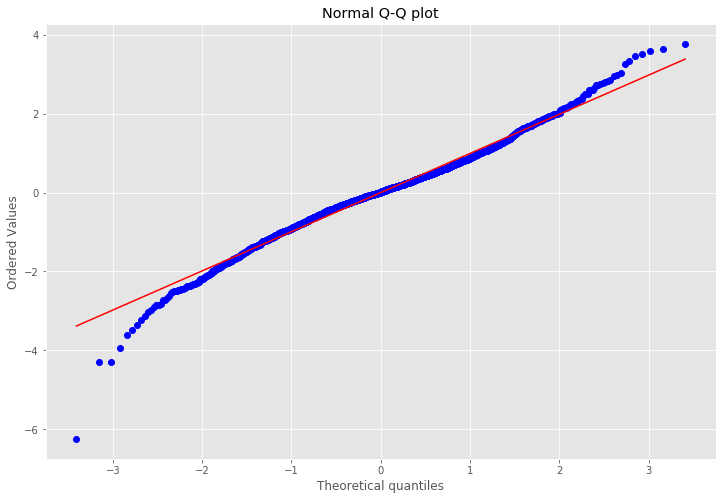The above Normal Q-Q plot indicates that the distribution of the log returns is heavy tailed.

### Kurtosis and Skewness¶

Kurtosis — Kurtosis is a measure of the combined weight of a distribution's tails relative to the center of the distribution. It esssentially tells us whether the data is heavy-tailed or light-tailed relative to a normal distribution. The kurtosis of any univariate normal distribution is 3. Distributions with kurtosis less than 3 are said to be platykurtic, while those with kurtosis greater than 3 are said to be leptokurtic. In python, the kurtosis can be calculated using the kurtosis function of pandas. This, however, calculates the excess Kurtosis(Kurtosis expressed as above or below 3), as it assumes the Fisher's definition of Kurtosis, whereby Kurtosis of Normal distribution is equal to 0.

Skewness — Skewness is a measure of symmetricity of the distribution and can be mathematically defined as the averaged cubed deviation from the mean divided by the standard deviation cubed. If the result of the computation is greater than zero, the distribution is positively skewed. If it's less than zero, it's negatively skewed. If equal to zero, it's roughly symmetric. Normal distrbution has a skewness measure of zero. The funtion skew of pandas can be used to calculate the skewness of a distribution.

In :
daily_return_excess_kurtosis = daily_log_returns[1:].kurtosis()
daily_return_excess_skewness = daily_log_returns[1:].skew()
print("Excess Kurtosis of distribution of {} daily returns is {:.3f}".format(ticker, daily_return_excess_kurtosis))
print("Skewness of distribution of {} daily returns is {:.3f}".format(ticker, daily_return_excess_skewness))
# p = stats.normaltest(daily_returns[1:])
# print(p1)
# print(p)

Excess Kurtosis of distribution of NSE/NIFTY_50 daily returns is 1.756
Skewness of distribution of NSE/NIFTY_50 daily returns is -0.195


### JB Test for Normality¶

The Jarque-Bera Test (JB Test) is a test for Normality. Specifically, the test matches the skewness and kurtosis of data to see if it matches a Normal distribution.

The JB test tests the hypothesis

Ho : Data is Normal

Ha : Data is NOT Normal

using the test statistic

\begin{equation*} JB_{TestStat} = n\left(\frac{\hat{Skewness}^2}{6} + \frac{\hat{ExcessKurtosis}^2}{24}\right) \quad\text{where n is the sample size} \end{equation*}

If data came from a Normal distriubtion, the test statistic would assume the value zero.

Note that this test only works for a large enough number of data samples (>2000) as the test statistic asymptotically has a Chi-squared distribution with 2 degrees of freedom.

The code given below calculates the JB test statistic and its corresponding p-value for the distribution using the function jarque_bera from scipy.stats

In :
JB_TestStat = len(daily_log_returns[1:])*((daily_return_excess_skewness**2)/6+(daily_return_excess_kurtosis**2)/24)
print("JB test statistic calculated for {} daily returns is {:.3f}".format(ticker,JB_TestStat))

JB_TestStat,JBpv = stats.jarque_bera(daily_log_returns[1:])
print("JB test statistic for {} daily returns is {:.3f}".format(ticker,JB_TestStat))
print("p-value for JB test for {} daily returns is {:.3f}".format(ticker,JBpv))

if JBpv>0.05:
print("The p-value of {} implies that we reject the alternate hypothesis of distribution of log returns of {} not being normal with 95% confidence level".format(JBpv,ticker))
else:
print("The p-value of {} implies that we reject the null hypothesis of distribution of log returns of {} being normal with 95% confidence level".format(JBpv,ticker))


JB test statistic calculated for NSE/NIFTY_50 daily returns is 284.384
JB test statistic for NSE/NIFTY_50 daily returns is 282.208
p-value for JB test for NSE/NIFTY_50 daily returns is 0.000
The p-value of 0.0 implies that we reject the null hypothesis of distribution of log returns of NSE/NIFTY_50 being normal with 95% confidence level


## 7. Heatmap and Seasonality¶

### Monthly Returns as a Heatmap¶

A heatmap is a graphical representation of matrix data where the individual values contained are represented as colors.

The code given below plots the monthly returns against their respective years in the form of a heatmap. It is done by first resampling the daily data as monthly, by taking the last published value of the month. Using these end of month levels, we calculate the monthly return, which is then grouped by 'Year' and 'Month'. The matrix hence obtained is plotted as a heatmap using the function heatmap of the library seaborn.

The colour scheme of the map highlights the high positive returns towards the green side of the spectrum, while high negative returns are towards the red side.

In :
nifty_levels_monthly = nifty_levels_test.resample('M').last()
nifty_monthly_returns = pd.DataFrame(index = nifty_levels_monthly.index[1:])
nifty_monthly_returns['Monthly Returns'] = nifty_levels_monthly['Close'].pct_change()
nifty_monthly_returns['Month'] = nifty_monthly_returns.index.strftime('%b')
nifty_monthly_returns['Year'] = nifty_monthly_returns.index.year
df = nifty_monthly_returns.groupby(['Year', 'Month'], sort=False)['Monthly Returns'].last()
df = df.unstack(level=0)
_ = sns.heatmap(df, annot=True, fmt=".2%", cmap="RdYlGn", center=0)
plt.rcParams['figure.figsize'] = [12, 8]
plt.title("Monthly Return Plot")
plt.show()